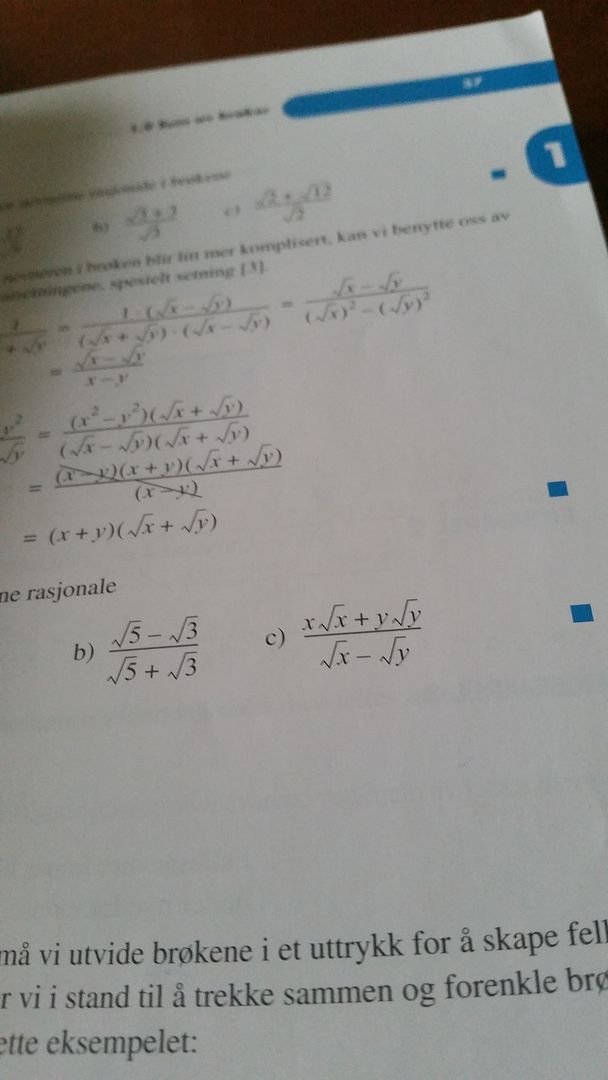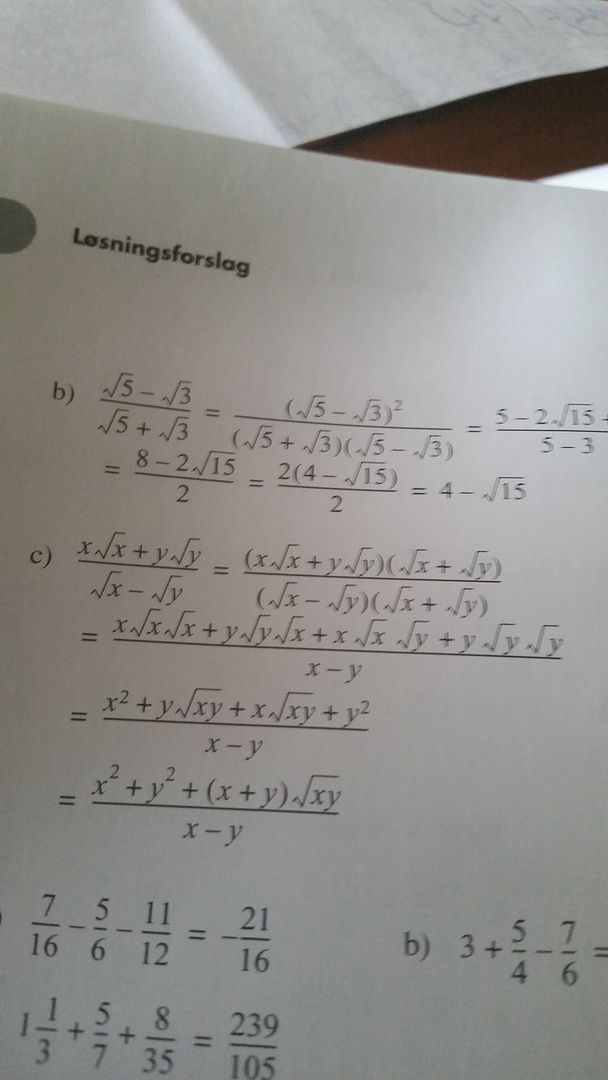User Name Remember Me? Password

 Algebra Pre-Algebra and Basic Algebra Math Forum

 May 12th, 2015, 06:25 AM #1 Member   Joined: Jan 2015 From: Norway Posts: 95 Thanks: 1 rationalize a denominator last task Task 1.25 C) This is the taskand this is the answer in the bookI do understand that (x squareroot x - y square root y) ( squareroot x - squaretoot y/(squareroot x)^2 - (squareroot y)^2 is x -y Also will be (x square root x + y square root y)^2 + (square root x - squareoot y)^2/ x -y I also understand that you use square sentence three in this example. But i don't know what happens after that and why. I am stuck in that part. Would be fine if someone could help me.May 12th, 2015, 07:26 AM #2 Senior Member   Joined: Apr 2014 From: Glasgow Posts: 2,157 Thanks: 732 Math Focus: Physics, mathematical modelling, numerical and computational solutions Maybe it will be easier to consider the denominator and numerator separately and show exactly what happens step by step. So, after multiplying the top and bottom by the conjugate of the denominator (i.e. $\displaystyle \sqrt{x} + \sqrt{y}$) Denominator: Start with: $\displaystyle (\sqrt{x} - \sqrt{y})(\sqrt{x} + \sqrt{y})$ Multiply out brackets: $\displaystyle =\sqrt{x}\sqrt{x} + \sqrt{y}\sqrt{x} - \sqrt{x}\sqrt{y} - \sqrt{y}\sqrt{y}$ The two middle terms subtract with each other. $\displaystyle =\sqrt{x}\sqrt{x} - \sqrt{y}\sqrt{y}$ Because $\displaystyle \sqrt{a}\sqrt{a} = \sqrt{a \times a} = \sqrt{a^2} = a$, the next step is to apply this to the x-part and the y-part: $\displaystyle =x -y$ Now for the numerator: Start with: $\displaystyle (x\sqrt{x} + y\sqrt{y})(\sqrt{x} + \sqrt{y})$ Multiply out the brackets: $\displaystyle =x\sqrt{x}\sqrt{x} + x\sqrt{y}\sqrt{x} + y\sqrt{x}\sqrt{y} - y\sqrt{y}\sqrt{y}$ Because $\displaystyle \sqrt{a}\sqrt{a} = \sqrt{a \times a} = \sqrt{a^2} = a$, the next step is to apply this to the first and last terms: $\displaystyle =x\times x + x\sqrt{y}\sqrt{x} + y\sqrt{x}\sqrt{y} + y \times y$ Use index notation (i.e. $\displaystyle a \times a = a^2$): $\displaystyle =x^2 + x\sqrt{y}\sqrt{x} + y\sqrt{x}\sqrt{y} + y^2$ Factorise $\displaystyle \sqrt{x}\sqrt{y}$ from the middle two terms: $\displaystyle =x^2 + \sqrt{y}\sqrt{x}(x+y) + y^2$ Rearrange order slightly: $\displaystyle =x^2 + y^2 + (x+y)\sqrt{y}\sqrt{x}$ Putting together, the final expression is: $\displaystyle \frac{x^2 + y^2 + (x+y)\sqrt{y}\sqrt{x}}{x-y}$May 12th, 2015, 03:55 PM   #3
Banned Camp

Joined: Jun 2014
From: Earth

Posts: 945
Thanks: 191

Quote:
 Originally Posted by Benit13Because $\displaystyle \sqrt{a}\sqrt{a} = \sqrt{a \times a} = \sqrt{a^2} = a$
That's not true.

$\displaystyle \sqrt{a}\sqrt{a} \ \ne \ \sqrt{a \times a}$

$\displaystyle \sqrt{a \times a} \ = \ \sqrt{a^2} \ = \ |a|$

It's because $\displaystyle \ \ \sqrt{a}\sqrt{a} \ = \ (\sqrt{a})^2 \ = \ a.$May 12th, 2015, 05:15 PM   #4
Senior Member

Joined: Jan 2014
From: The backwoods of Northern Ontario

Posts: 391
Thanks: 70

Quote:
 Originally Posted by Math Board Tutor That's not true. $\displaystyle \sqrt{a}\sqrt{a} \ \ne \ \sqrt{a \times a}$
I don't understand. Could you provide a positive value of "a" where the two sides are not equivalent?May 13th, 2015, 11:26 AM   #5
Banned Camp

Joined: Jun 2014
From: Earth

Posts: 945
Thanks: 191

Quote:
 Originally Posted by TimiosI don't understand. Could you provide a positive value of "a" where the two sides are not equivalent?
Quote:
 Originally Posted by Math Message Board tutorThat's not true. $\displaystyle \sqrt{a}\sqrt{a} \ \ne \ \sqrt{a \times a}$
No, it's that the two sides are not equal in general.

Example:

$\displaystyle (\sqrt{-1})(\sqrt{-1}) \ = \ (i)(i) \ = \ i^2 \ = \ -1 \ \ne \ \sqrt{(-1)(-1) \ } \ = \ \sqrt{ \ 1 \ } \ = \ 1$

Last edited by skipjack; November 8th, 2015 at 04:57 PM.May 13th, 2015, 12:39 PM #6 Math Team   Joined: Dec 2013 From: Colombia Posts: 7,675 Thanks: 2654 Math Focus: Mainly analysis and algebra It's true in the real numbers where this question obviously resides. MMBt is trying to find errors at the expense of confusing you. Unless you are working with complex numbers (if you don't know whether you are or not, then you aren't), $\sqrt{a} \times \sqrt{a} = \sqrt{a \times a}$ when $a \ge 0$. In the context of this example, $x$ and $y$ are clearly greater than or equal to zero since $\sqrt x$ and $\sqrt y$ both appear on the left hand side, so they must exist.May 16th, 2015, 03:54 PM   #7
Banned Camp

Joined: Jun 2014
From: Earth

Posts: 945
Thanks: 191

Quote:
 Originally Posted by v8archieIt's true in the real numbers where this question obviously resides. MMBt is trying to find errors at the expense of confusing you.
v8archie, you are passing off ignorance on this thread as some little child.
I corrected another tutor.

When I make a correction to another tutor about a point, you typed
a lie such as the above.

And think next time, "Gee, the OP didn't make a post referencing what
Math Message Board tutor stated. I won't assume then. I won't fix
something for which there's nothing broken then."

Summation: Get on about your business.

Last edited by Math Message Board tutor; May 16th, 2015 at 04:05 PM.May 18th, 2015, 01:47 AM   #8
Senior Member

Joined: Apr 2014
From: Glasgow

Posts: 2,157
Thanks: 732

Math Focus: Physics, mathematical modelling, numerical and computational solutions
Quote:
 Originally Posted by Math Message Board tutorv8archie, you are passing off ignorance on this thread as some little child. I corrected another tutor.
I'm not a tutor. Just in case you aren't aware, this isn't a tutoring website. It's a maths forum.

Quote:
 When I make a correction to another tutor about a point, you typed a lie such as the above.
I agree with V8Archie on this one.

I agree that $\displaystyle \sqrt{a \times a} \neq \sqrt{a}\sqrt{a}$ if $\displaystyle a$ is negative, as you stated. However, I'm not in the habit of constantly writing absolutely everything down in absolute fine detail just to avoid nitpicking details from pedants.

Last edited by Benit13; May 18th, 2015 at 02:02 AM.May 18th, 2015, 05:28 AM #9 Senior Member   Joined: Apr 2014 From: Europa Posts: 584 Thanks: 177 Let's admit, tacitly, that we are in the real world. So we have here: $\displaystyle \sqrt{a}\sqrt{a} = (\sqrt{a})^2 = a \\\;\\ \sqrt{a^2} = |a|, \forall\ a\in\mathbb{R}$May 18th, 2015, 06:30 AM   #10
Senior Member

Joined: Apr 2014
From: Europa

Posts: 584
Thanks: 177

Quote:
 Originally Posted by Zman15I do understand that (x squareroot x - y square root y) ( squareroot x - squaretoot y/(squareroot x)^2 - (squareroot y)^2 is x -y Also will be (x square root x + y square root y)^2 + (square root x - squareoot y)^2/ x -y I also understand that you use square sentence three in this example.
Here is, exactly where it would have its place, a simple algebraic expression:

$\displaystyle E= \dfrac{x\sqrt{x}+y\sqrt{y}}{\sqrt{x}-\sqrt{y}}\ \Longrightarrow\ E = \dfrac{(\sqrt{x})^3+(\sqrt{y})^3}{\sqrt{x}-\sqrt{y}}$

Let's write:

$\displaystyle \sqrt{x}=a,\ \ \ \sqrt{y}=b$

Now, we have:

$\displaystyle E=\dfrac{a^3+b^3}{a-b}$

Amplify with a+b.Tags denominator, rationalize, taskThread ToolsShow Printable VersionEmail this Page Display ModesLinear ModeSwitch to Hybrid ModeSwitch to Threaded ModeSimilar Threads Thread Thread Starter Forum Replies Last Post Zman15 Algebra 2 May 10th, 2015 10:43 AM Stingray Calculus 13 September 21st, 2014 05:06 PM tmtwd Algebra 1 April 9th, 2013 12:12 PM tallbabe1 Algebra 6 December 14th, 2012 05:57 PM icantsolvethese Algebra 3 August 29th, 2012 03:04 PM

 Contact - Home - Forums - Cryptocurrency Forum - Top

Copyright © 2019 My Math Forum. All rights reserved.